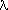# Wind Measurement

The meridional velocity is the distance, r ·, divided by the time difference,t. Since the radius is given in Km's and the time is given in hours we need factors of 1000 and 3600, respectively, to convert to meters per second. Ifis given in degrees then:

V = 1000 R() · (/180)(1 -0)/(3600t)

To calculate the zonal velocity we need to calculate the displacement along a latitude circle, (i.e.,/ 360), during the time intervalt.=(t1) -(t0)

U = 2R(<>) cos(<>) · (1000/3600) ·/(360t)

Alternatively, we can calculate the period of rotation of the measured feature, P, and define the wind relative to the system of measure, Pref as follows:

P = 360t/[+ (360t/Pref) ]

so that,

U = 2R(<>) cos(<>) · (1000/3600) · ( 1/P - 1/Pref )